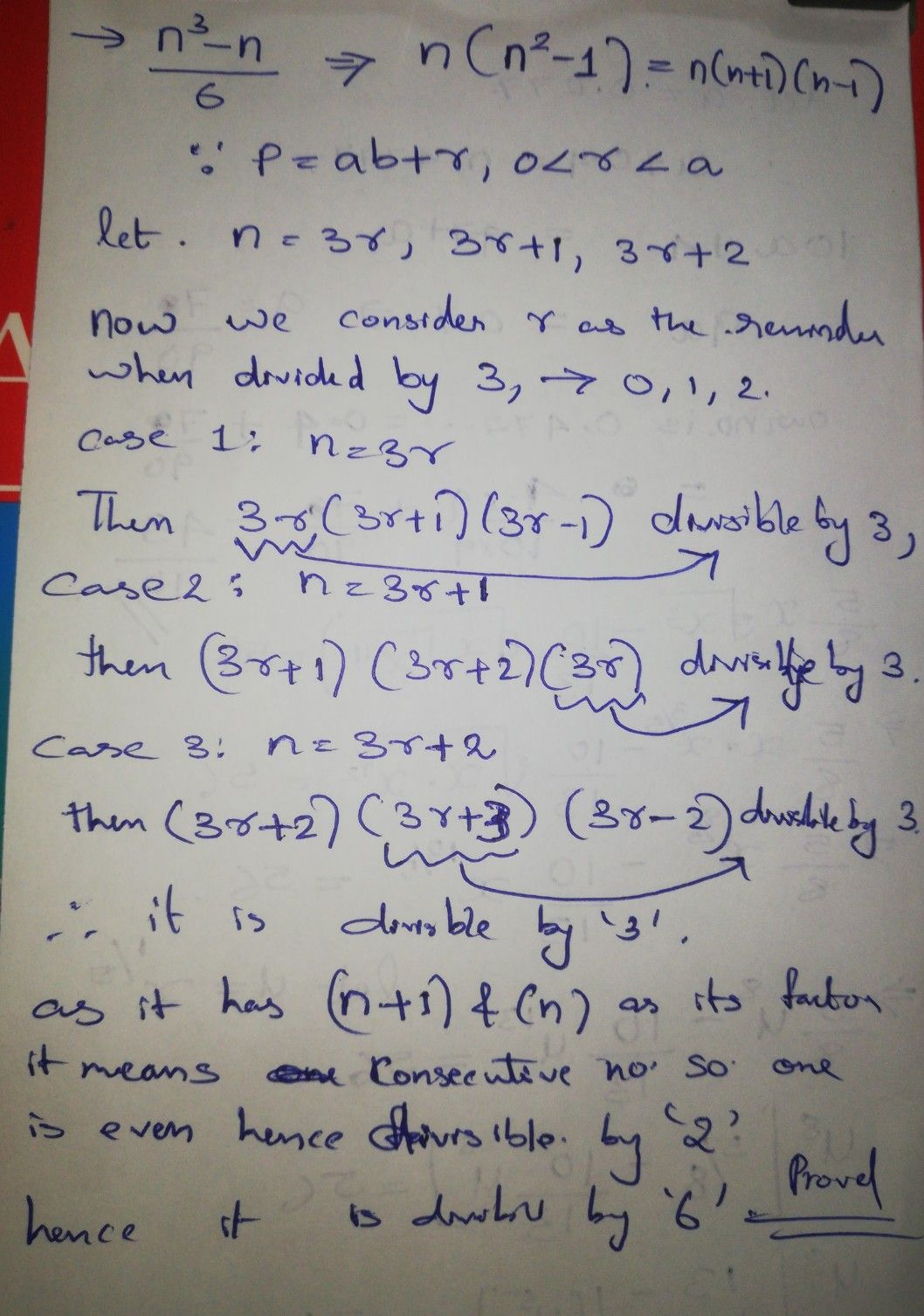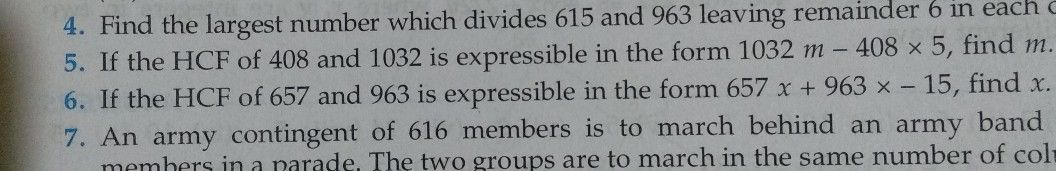Symbol
Problem$4$ $Q3$ Prove that the product of three consecutive positive integer is div $0$ $40$ For any positive integer $n,$ prove that $n^{3}-n$ divisible by $6.$ $2$ $5$ Prove that if a positive integer is of the form $6q+5,$ then it is of the
10th-13th grade
Other
SolutionQanda teacher - Ajith Kuma
Harsh which question you want me to do, is it the 4 th question answer me !!Student
yes sir 4th oneQanda teacher - Ajith Kuma
yes I'm working on it!Student
thanku sir?Qanda teacher - Ajith Kumaif you understand my solution, care to rate it.
I hope I have answered ur question , if you have any queries regarding the solution feel free to ask. Have a great day.Student
thanks sir
sir can i ask u one more question ?
pleade
please*Qanda teacher - Ajith Kuma
if you have any queries I can answer , if want to ask more ask in 1-1 sections.
Thank youStudent
but sir i have no more coupons leftQanda teacher - Ajith Kuma
lemme c , wat is it
I will answer one more!!Student
thanku sir5th one
Sir I have asked u this type of question in one more chat?Qanda teacher - Ajith Kuma
regarding this question talk, other chat talk thereStudent
ok sir
can you please answer me that question ?
there in that talk